# 25 JavaScript Tricks You Need To Know About (Part 2)

## 1 — Deep value retriever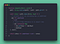Code on Github
`deepValue(obj, 'top.in.list.0'); // get list 1st itemdeepValue(obj, 'top.in.list.length'); // get list lengthdeepValue(obj, 'top.in.noExistentKey'); // returns null`

## 2 — Date Formatter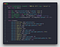Code on Github
`formatDate(Date.now(), 'MM-DD-YYYY'); // "02-06-2021"formatDate(Date.now()); // "February 06, 2021"formatDate(Date.now(), 'D de MMMM de YYYY', 'pt'); // "6 de fevereiro de 2021"formatDate(Date.now(), 'MMMM DD, YYYY', 'zh'); // "二月 06, 2021"formatDate(Date.now(), 'MMM DD (DDD), YYYY'); // "Feb 06 (Sat), 2021"`

## 3 — Promisifier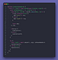Code on Github
`const add = (a, b) => { return a + b;}const divide = (a, b, cb) => {  if(b === 0 && a !== 0) {    cb(new Error('Cannot divide by "Zero"'), null)  } else {    const total = a / b;    cb(null, total);  }}const asyncAdd = promisify(add);const asyncDivide = promisify(divide);add(23, 67); // 90asyncAdd(23, 67).then(total => {  console.log(total); // 90});divide(35, 5, (error, total) => {  console.log(error) // null  console.log(total) // 7});divide(8, 0, (error, total) => {  console.log(error) // Error: 'Cannot divide by "Zero"'  console.log(total) // 'total' null});asyncDivide(35, 5)  .then((total) => {    console.log(total); // 7  });asyncDivide(35, 0)  .catch((error) => {    console.log(error); // Error: 'Cannot divide by "Zero"'  });`

## 4 — Merge Objects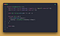Code on Github

## 5 — Deep Equality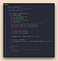Code on Github

## 6 — Siblings selector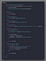Code on Github
`element('ul li:nth-of-child(3)').siblingselement('.item-3').previousSiblingselement(document.getElementById('section-4')).nextSiblings`

## 7 — Ancestor selector

`<section id="top">  <div><span>text</span></div>  <form action="">    <fieldset>      <label for="">        <input type="">      </label>    </fieldset>  </form></section>const input = document.querySelector('input');input.parentElement // "<label for=''>...</label>"input.closest('form') // "<form action=''>...</form>"input.closest('#top') // "<section id='top'>...</section>"`

## 8 — Key list by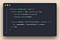Code on Github
`const arr = [  {id: 1, name: 'one'},  {id: 2, name: 'two'},  {id: 3, name: 'three'},];keyBy(arr, 'id');/* outputs {  '1': { id: 1, name: 'one' },  '2': { id: 2, name: 'two' },  '3': { id: 3, name: 'three' }}*/const obj = {  1: {id: 1, str: 'uno'},  2: {id: 2, str: 'doz'},  3: {id: 3, str: 'tres'}}keyBy(obj, 'str');/* outputs{  uno: { id: 1, str: 'uno' },  doz: { id: 2, str: 'doz' },  tres: { id: 3, str: 'tres' }}*/const map = new Map([  ['one', {id: 1, name: 'one'}],  ['two', {id: 2, name: 'two'}],  ['three', {id: 3, name: 'three'}]]);keyBy(map, 'name');/* outputs{  one: { id: 1, name: 'one' },  two: { id: 2, name: 'two' },  three: { id: 3, name: 'three' }}*/`

## 9 — Loop Anything with the ability to break out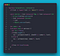Code on Github
`forEach([1, 2, 3], console.log) // arrayforEach('123', console.log) // stringforEach({a: 1, b: 3, c: 4}, console.log) // objectforEach(new Map([['a', 1], ['b', 20]]), console.log) // MapforEach(new Set([4, 12, 89]), console.log) // SetforEach(new Set([4, 12, 89]).keys(), console.log) // Iterator`

## 12 — Map Anything

`const handler = n => n + 10;map([1, 2, 3], handler) // arraymap('123', handler) // stringmap({a: 1, b: 3, c: 4}, handler) // objectmap(new Map([['a', 1], ['b', 20]]), handler) // Mapmap(new Set([4, 12, 89]), handler) // Setmap(new Set([4, 12, 89]).keys(), handler) // Iterator`

## 11 — Filter anything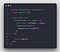Code on Github
`const arr = [2, 4, 7, 13, 89];filter(arr, (n) => n > 7) // returns [ 13, 89 ]`

## 12 — Fixed Size Array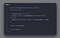Code on Github
`const arr1 = createFixedSizeArray(10, 2, 3, 4);const arr2 = createFixedSizeArray(3);arr1 = 20; // has no effectarr1.push(10) // throws TypeError: Cannot add property...arr1.pop() // throws TypeError: Cannot delete property ...arr2 = 12; // sets the value since index one slot existsconsole.log(arr1); // prints [ 10, 2, 3, 4 ]console.log(arr2); // prints [ undefined, 12, undefined ]`

## 13 — Capitalize Words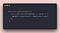Code on Github
`capitalize("My dog ate my homework") // returns 'My Dog Ate My Homework'`

## 12 — File Uploader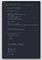Code on Github
`postData('http://localhost:1234/upload', {name: 'John Doe'})  .then(res => {    console.log('end', res)  });const onProgress = event => {  console.log(event.total, event,loaded);}postData('http://localhost:1234/upload', myFile, {onProgress})`

## 15 — Find Average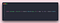Code on Github

## 16 — Group Data by key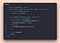Code on Github
`const people = [  {firstName: 'John', lastName: 'Doe'},  {firstName: 'Peter', lastName: 'Carter'},  {firstName: 'Jane', lastName: 'Sigfield'},  {firstName: 'Jonathan', lastName: 'Sigfield'},  {firstName: 'Alice', lastName: 'Doe'},  {firstName: 'Carlos', lastName: 'Carter'},  {firstName: 'Bruno', lastName: 'Sigfield'},  {firstName: 'Before', lastName: 'Semicolon'}];groupBy(people, 'lastName')/* outputs{  Doe: [    { firstName: 'John', lastName: 'Doe' },    { firstName: 'Alice', lastName: 'Doe' }  ],  Carter: [    { firstName: 'Peter', lastName: 'Carter' },    { firstName: 'Carlos', lastName: 'Carter' }  ],  Sigfield: [    { firstName: 'Jane', lastName: 'Sigfield' },    { firstName: 'Jonathan', lastName: 'Sigfield' },    { firstName: 'Bruno', lastName: 'Sigfield' }  ],  Semicolon: [ { firstName: 'Before', lastName: 'Semicolon' } ]}*/`

## 20 — Debounce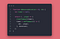Code on Github
`const btn = document.querySelector('button');const clickHandler = event => {  console.log(event.target)}btn.addEventListener('click', debounce(1000, clickHandler));`

## 21 — Handle Event Once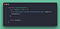Code on Gtihub
`const btn = document.querySelector("button");btn.addEventListener("click", once(console.log));`

## 22 — Handle Event until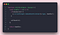Code on Github
`const btn = document.querySelector("button");const handler = e => {  const n = Number(e.currentTarget.textContent);  e.currentTarget.textContent = n + 1;}const checker = e => Number(e.currentTarget.textContent) >= 5;btn.addEventListener("click", until(handler, checker));`

## 23 — Observe DOM element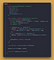Code on Github
`const btn = document.querySelector('button');observeElement(btn, console.log);btn.style.display = 'block';/* triggers{  type: "attributes",   oldValue: null,   newValue: "display: block;"}*/btn.innerHTML = 'another text <span>label</span> after';/* triggers{  type: "content",   oldValue: null,   newValue: ["another text ", span, " after"]}*/`

## 24 —Simple Observable (like RxJs)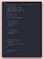Code on Github
`const obs = new Observable((observer) => {  observer.next(10);  observer.next(20);  observer.complete();  // gets ignored since its complete  observer.error(new Error()); })obs.subscribe({  next(value) {    console.log(value); // prints 10, 20  }});obs.unsubscribe();// never executesobs.subscribe((value) => {  console.log('next', value);});`

## 25 — Extend DOM element with data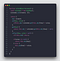Code on Github
`let btn = extendObject(document.querySelector('button'));let array = extendObject([1, 2, 100]);// extends API with "position"btn.set('position', (() => btn.obj.getBoundingClientRect())());btn.get('position') // {x: 8, y: 8, width: 34.53125, height: 21, top: 8, …}// Add ability to get last item to arrayarray.set('lastItem', (() => array.obj.slice(-1))());array.get('lastItem') // 100`

## Conclusion

Blog & YouTube Channel for Web, UI & Software Development - beforesemicolon.comyoutube.com/c/BeforeSemicolon

## More from Before Semicolon

Blog & YouTube Channel for Web, UI & Software Development - beforesemicolon.com — youtube.com/c/BeforeSemicolon